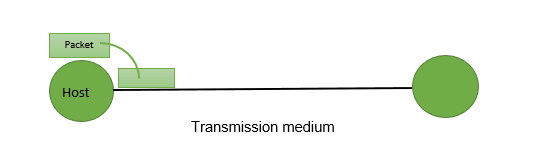Open in App
Not now

# Delays in Computer Network

• Difficulty Level : Basic
• Last Updated : 27 Sep, 2021

The delays, here, means the time for which the processing of a particular packet takes place. We have the following types of delays in computer networks:

1. Transmission Delay:
The time taken to transmit a packet from the host to the transmission medium is called Transmission delay.For example, if bandwidth is 1 bps (every second 1 bit can be transmitted onto the transmission medium) and data size is 20 bits then what is the transmission delay? If in one second, 1 bit can be transmitted. To transmit 20 bits, 20 seconds would be required.

Let B bps is the bandwidth and L bit is the size of the data then transmission delay is,

`Tt = L/B`

This delay depends upon the following factors:

• If there are multiple active sessions, the delay will become significant.
• Increasing bandwidth decreases transmission delay.
• MAC protocol largely influences the delay if the link is shared among multiple devices.
• Sending and receiving a packet involves a context switch in the operating system, which takes a finite time.

2. Propagation delay:
After the packet is transmitted to the transmission medium, it has to go through the medium to reach the destination. Hence the time taken by the last bit of the packet to reach the destination is called propagation delay.Factors affecting propagation delay:

1. Distance – It takes more time to reach the destination if the distance of the medium is longer.
2. Velocity – If the velocity(speed) of the signal is higher, the packet will be received faster.
`Tp = Distance / Velocity `

Note:

```Velocity =3 X 10^8 m/s (for air)
Velocity= 2.1 X 10^8 m/s (for optical fibre) ```

3. Queueing delay:
Let the packet is received by the destination, the packet will not be processed by the destination immediately. It has to wait in a queue in something called a buffer. So the amount of time it waits in queue before being processed is called queueing delay.

In general, we can’t calculate queueing delay because we don’t have any formula for that.

This delay depends upon the following factors:

• If the size of the queue is large, the queuing delay will be huge. If the queue is empty there will be less or no delay.
• If more packets are arriving in a short or no time interval, queuing delay will be large.
• The less the number of servers/links, the greater is the queuing delay.

4. Processing delay:
Now the packet will be taken for the processing which is called processing delay.

Time is taken to process the data packet by the processor that is the time required by intermediate routers to decide where to forward the packet, update TTL, perform header checksum calculations.

It also doesn’t have any formula since it depends upon the speed of the processor and the speed of the processor varies from computer to computer.

Note: Both queueing delay and processing delay doesn’t have any formula because they depend on the speed of the processor
This delay depends upon the following factors:

• It depends on the speed of the processor.
```Ttotal = Tt + Tp + Tq + Tpro

Ttotal = Tt+Tp
(when taking Tq and Tpro equals to 0) ```

My Personal Notes arrow_drop_up